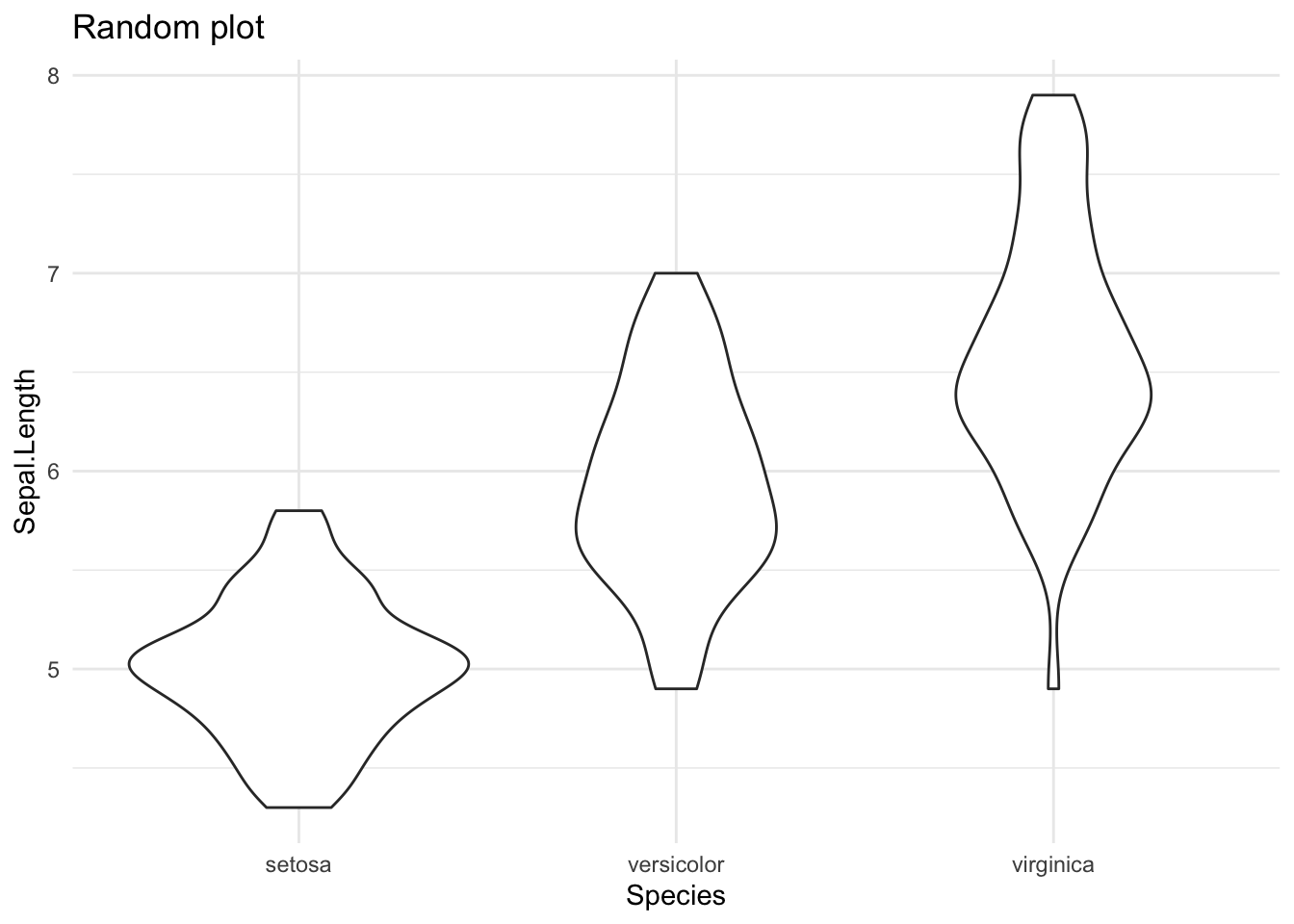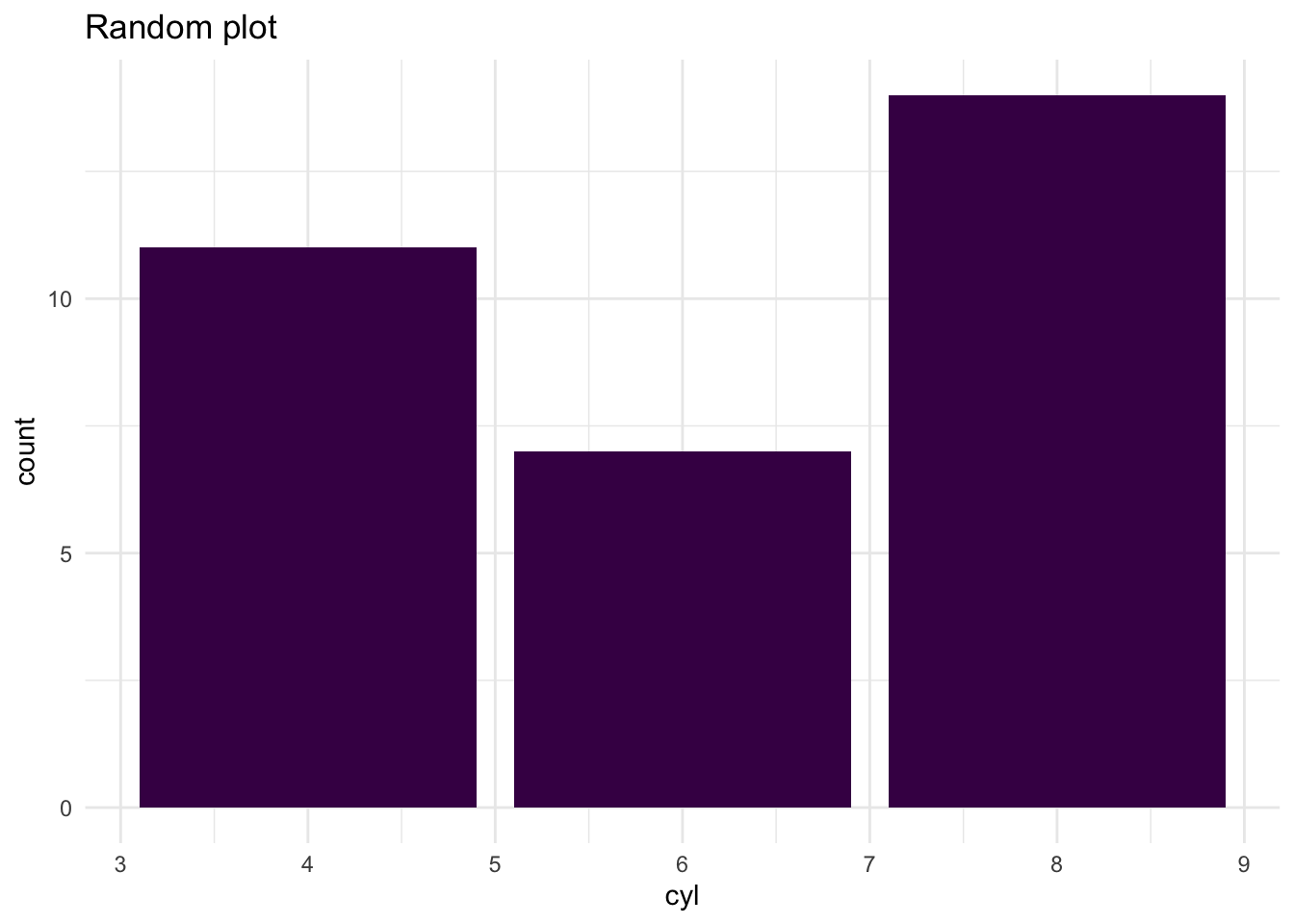# {shinipsum} is now on CRAN

Author

colin

Published

May 10, 2020I’m very happy to announce that `{shinipsum}` is now on CRAN!

`{shinipsum}` is a package that can help you build `{shiny}` prototypes faster by providing a series of functions that can generate random elements to populate your UI. If you are familiar with “lorem ipsum”, the fake text generator that is used in software design as a placeholder for text, the idea is the same: generating placeholders for Shiny outputs.

`{shinipsum}` can be installed from CRAN with:

``install.packages("shinipsum")``

You can install this package from GitHub with:

``remotes::install_github("Thinkr-open/shinipsum")``

In this package, a series of functions that generates random placeholders. For example, `random_ggplot()` generates random `{ggplot2}` elements:

``````library(shinipsum)
library(ggplot2)``````
``````random_ggplot() +
labs(title = "Random plot")````````````random_ggplot() +
labs(title = "Random plot")``````Of course, the idea is to combine this with a Shiny interface, for example `random_ggplot()` will be used with a `renderPlot()` and `plotOutput()`. And as we want to prototype but still be close to what your final application will look like, these functions take arguments that can shape the output: for example, `random_ggplot()` has a `type` parameter that can help you select a specific geom.

``````library(shiny)
library(shinipsum)
library(DT)
ui <- fluidPage(
h2("A Random DT"),
DTOutput("data_table"),
h2("A Random Plot"),
plotOutput("plot"),
h2("A Random Text"),
tableOutput("text")
)

server <- function(input, output, session) {
output\$data_table <- DT::renderDT({
random_DT(5, 5)
})
output\$plot <- renderPlot({
random_ggplot(type = "point")
})
output\$text <- renderText({
random_text(nwords = 50)
})
}
shinyApp(ui, server)``````

Other `{shinipsum}` functions include:

• tables:
``random_table(nrow = 3, ncol = 10)``
``````  Plant   Type  Treatment conc uptake Plant.1 Type.1 Treatment.1 conc.1
1   Qn1 Quebec nonchilled   95   16.0     Qn1 Quebec  nonchilled     95
2   Qn1 Quebec nonchilled  175   30.4     Qn1 Quebec  nonchilled    175
3   Qn1 Quebec nonchilled  250   34.8     Qn1 Quebec  nonchilled    250
uptake.1
1     16.0
2     30.4
3     34.8``````
• print outputs:
``random_print(type = "model")``
``````
Pearson's product-moment correlation

data:  datasets::mtcars\$mpg and datasets::mtcars\$cyl
t = -8.9197, df = 30, p-value = 6.113e-10
alternative hypothesis: true correlation is not equal to 0
95 percent confidence interval:
-0.9257694 -0.7163171
sample estimates:
cor
-0.852162 ``````

… and text, image, ggplotly, dygraph, and DT.

Learn more about `{shinipsum}`: